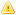Home |Sign in | EnglishHelpWLanguageWLanguage functionsStandard functionsNumeric values management functionsFinancial functionsFinFutureValPresentationExampleTime unitManaging errorsRelated examplesSee alsoFinCurrentValFinDecreasingRedemptionFinErrorFinFutureValFinInterestRateFinLinearRedemptionFinNetCurrentValFinPaymentNbFinPeriodInterestFinRedemptionFinRepayValFinancial constantsFinancial functionsThis content has been translated automatically. Click here to view the French version.
• Time unit
• Managing errors
WINDEVWEBDEVWINDEV MobileOthersFinFutureVal (Function)
In french: FinValFuture
Returns the future value of an investment. The payments are regular and fixed. The interest rate is fixed.Versions 23 and laterThis function is now available for Java applications.New in version 23This function is now available for Java applications.This function is now available for Java applications.
// Future value of an investment. The initial capital is set to 100000 Euros.
// 3000 Euros are paid each month during 2 years. The interest rate is set to 8%.
ResFutureVal = FinFutureVal(-100000, -3000, 24, 8)
Syntax
<Result> = FinFutureVal([<Initial Capital> [, <Periodic Deposit> [, <Number of Periods> [, <Rate> [, <Start>]]]]])
<Result>: Real or currency
Future value of the investment.
<Initial Capital>: Optional real or currency
Initial capital (0 by default).
<Periodic Deposit>: Optional real or currency
Periodic deposit (0 by default). This value is fixed for the entire duration of the operation. In most cases, this amount includes the capital and the interests.
<Number of Periods>: Optional integer
Total number of periods for the operation (1 by default).
<Rate>: Optional real
Interest rate of investment expressed in percentage (1 by default). For example, for a 5% rate, this parameter will be set to 5. This rate cannot be null.
<Start>: Optional boolean
• True if the payments must be made at the beginning of period (default value),
• False if the payments must be made at the end of period.
Remarks

Time unit

The calculations can be performed according to months or years. <Rate> and <Period> must correspond to the same unit.
Therefore, for monthly payments of a 5-year loan with a yearly interest rate set to 8%, use:
• <Rate> = 8% / 12,
• <Number of Periods> = 5*12
For the same loan with yearly payments, use:
• <Taux> = 8%,
• <Number of Periods> = 5
For all the parameters, the payments are represented by a negative number while the collections are represented by a positive number.

Managing errors

Caution: FinFutureVal returns no error code. To find out whether errors have been generated by this function, use FinError. To find out the error details, use ErrorInfo associated with the errMessage constant.
Related Examples:Unit examples (WINDEV): The financial functions [ + ] Using the main functions for performing financial calculations in WINDEV:- Calculate the number of repayments- Calculate the future value of an investmentComplete examples (WINDEV): WD Loan [ + ] The power of WINDEV and the ease-of-use of WLanguage have allowed us to develop this application within a single day!This example is used to simulate loans and to:- calculate the amount of the monthly repayments from the amount borrowed- calculate the amount that can be borrowed from a given monthly repayment- calculate the income of an investment from a monthly paymentFor each case, you have the ability to view the amortization table corresponding to your parameters.Unit examples (WEBDEV): The financial functions [ + ] This example presents the main functions for financial calculations of WEBDEV and it allows you to:- Calculate the number of reimbursements- Calculate the future value of an investmentUnit examples (WINDEV Mobile): The Financial functions [ + ] Use of financial functions.This example calculates the number of payments needed to pay back a loan, the total cost of a loan as well as the interests returned by an investment.
Component: wd260mat.dll
Minimum version required
• Version 9